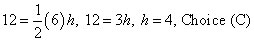##### GED Mathematical Reasoning Test For Dummies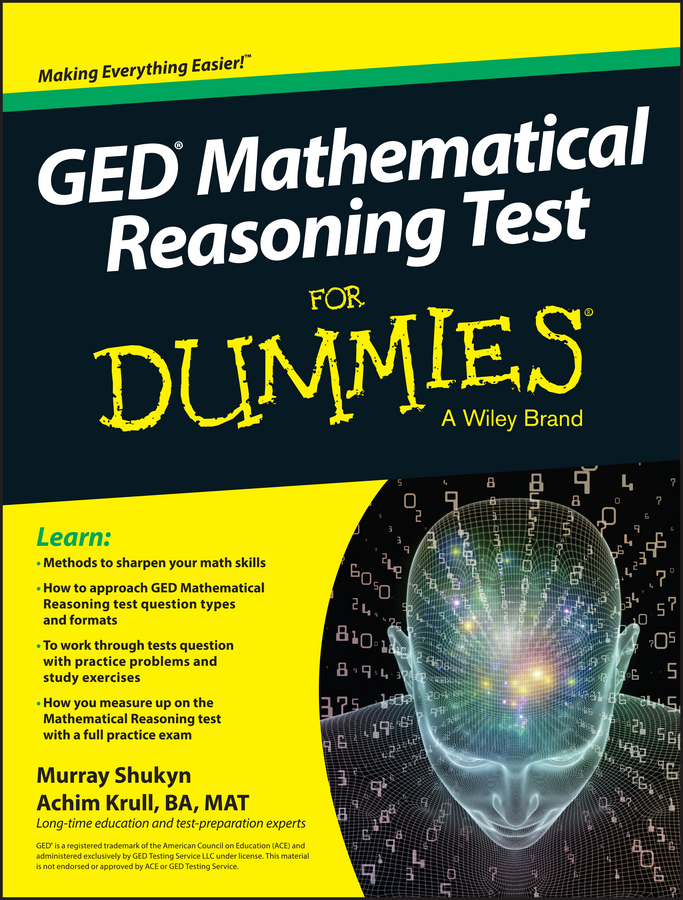The PSAT/NMSQT loves triangles, so you need to develop a little affection for them also. Fortunately, triangles are easy to love. Here are the facts about triangles:

• The angles inside a triangle add up to 180°. If you know two angles, you can figure out the third. Note: This fact appears in the information box on the exam.

• The largest angle is opposite the longest side of the triangle. Can you guess what else is true? The smallest angle is opposite the smallest side of the triangle.

• Sides of equal length are opposite equal angles. So if you have two sides, each of which is x in length, and opposite one of those sides is an angle that measures 45°, then the angle opposite the other side (that’s also x in length) will measure 45° as well.

• The sum of two sides must be greater than the length of the third side. If two sides of the triangle measure 4 and 6, the third side must be less than 10. This is the triangle inequality rule.

• The sides of similar triangles are in proportion. If you see a question referring to similar triangles, use your ratio skills to figure out the length of a side.

For example, suppose two similar triangles are in a ratio of 3:4, with the longest side of the smaller triangle measuring 30 meters. The longest side of the larger triangle is therefore 40 meters. The heights and bases of similar triangles are also in proportion.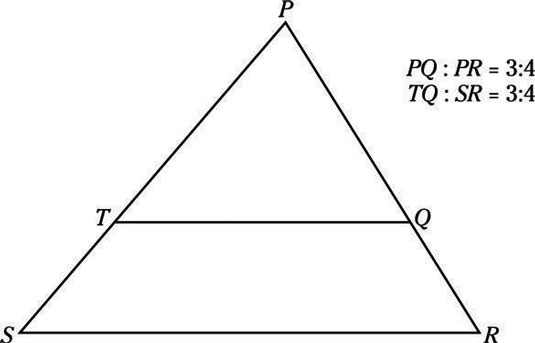• The ratio of the area of similar triangles equals the square of the ratio of their sides. If each side of triangle ABC is 1/2 the length of each side of triangle DEF, the area of triangle ABC is 1/4 of the area of triangle DEF, because (1/2)2 = 1/4.

The rules for similarity work for other shapes also, as long as their angles are equal and their sides are in proportion (angle to angle, side to side).

Remember that the diagrams on the PSAT/NMSQT can fool you. Unless the question says that the shapes are similar or you see that the triangles share angles, assume that the shapes you see aren’t similar.

• The area of a triangle = 1/2 base x the height. Note: This formula is in the information box on the exam. The height of a triangle (also known as the altitude) may be a side (in a right triangle) or it may be a line drawn perpendicular (at right angles) to the base of the triangle from the angle opposite the base.

• Or, in extremely rare and strange problems, the height may be outside the triangle, in which case it’s drawn as a broken line. In this figure, h is the height of each triangle. Notice the little square that indicates a right angle.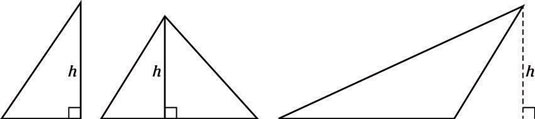Time to road-test these ideas. Try these four problems, all dealing with triangles.

1. In the following figure, triangle BCD is similar to triangle ACE, and the ratio of the length of AB to BC is 1:2. If the area of triangle BCD is 8, then what is the area of triangle ACE?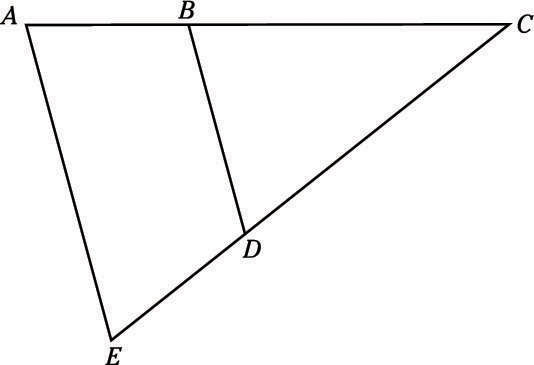(A) 10
(B) 12
(C) 14
(D) 16
(E) 18
2. Two sides of a triangle are 3 and 5 units long. Which of the following can’t be the length of the third side?

(A) 2
(B) 3
(C) 4
(D) 5
(E) 6
3. What is the perimeter of triangle ABC?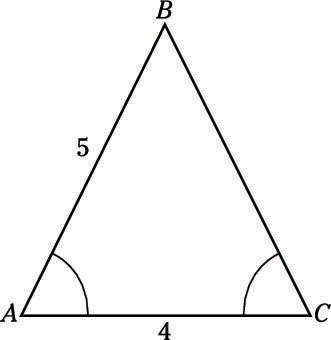(A) 7
(B)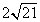(C) 14
(D)(E) 21
4. If the area of triangle ACD is 12, and the length of side AC is 6, what is the length of segment BD?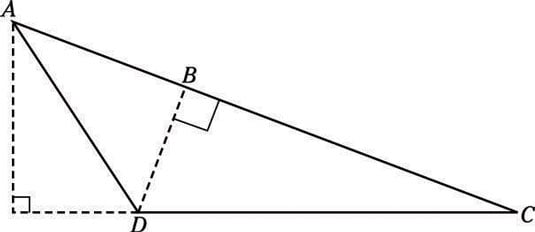(A) 2
(B) 3
(C) 4
(D) 5
(E) 6

1. E. 18

Remember that the ratio of the area of similar triangles is the square of the ratio of the lengths. Make sure that you notice the trick here: You’re given the ratio of AB to BC, not BC to AC. It’s easy to figure out the correct ratio, but if you miss that detail, you’ll be derailed.

BC:AC = 2:3, so the area ratio is 4:9. If the smaller triangle has an area of 8, then the larger triangle has an area of 18 (so that the ratio reduces from 8:18 to 4:9). Choice (E) is the one you want.

2. A. 2

This one is easy if you know the triangle inequality rule: “The sum of two sides must be greater than the length of the third side.” At first glance, all you know is that the third side must be shorter than 3 + 5 = 8 units long, but that fact doesn’t narrow down the answer choices, because none of them are too long.

So you have to look for side lengths that are too short. Because you’re trying to find something that’s too small, start by plugging in Choice (A). If the third side were 2 units long, then 2 + 3 = 5, but the other side is 5 units long, so 2 is not long enough! Choice (A) is correct.

3. C. 14

Angles A and C are equal, meaning that triangle ABC is an isosceles triangle. That means that side AB is the same length as side BC, so the perimeter of the triangle is 4 + 5 + 5 = 14, Choice (C).

4. C. 4

The trick to this problem is that you can look at segment AC as the base of the triangle and BD as the height. After you’ve got that figured out, you’re most of the way to the correct answer. Remember that the area equals 1/2bh, so you can plug everything into that equation and solve for h: